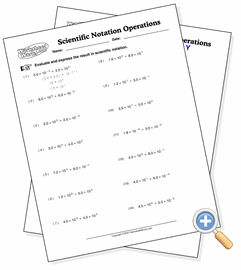# Scientific Notation OperationsDevelop skills in working with scientific numbers

This worksheet provides practice in multiplying numbers that are in scientific notation. While it is possible to convert the numbers to decimal form and multiply them, the correct way to do it is to multiply the decimal and exponent parts separately. Students will need to understand that multiplying two exponential powers with the same root means that the exponents should be added, or subtracted in the case of division.